# Convolutional Neural Networks Part 1¶

In :
from ipypublish import nb_setup


## Introduction¶

Convolutional Neural Networks or ConvNets were originally invented to do image processing. Their origin lies in the research done by Hubel and Wiesel in the 1960s on the visual perception systems in cats, which later won them a Nobel Prize (see 1959; 1962; 1968). Fukushima was inspired by their work and created a neural network model which he called the NeoCognitron, see Fukushima (1975); Fukushima (1980). This model was very similar model to modern ConvNets in its structure, however it lacked an efficient training algorithm, such as Backprop. LeCun, Bottou, Bengio, Haffner (1998) later put the two pieces of the puzzle together while working at Bell Labs during the 90s, and created the modern ConvNet, whose design was based on the NeoCognitron, and which could also be trained using Backprop. LeCun's system, called LeNet5, was successfully commercially deployed to read handwritten signatures in checks. Research in ConvNets lay dormant until 2012, when they were used for the winning entry in the ImageNet Large Scale Visual Recognition Competition (ILSVRC), see ILSVRC (2015). This also coincided with a resurgence of interest in neural networks, and since then ConvNets have led the field as the technology behind some of the most powerful DLNs.

## Why Dense Feed Forward Networks don't work well for Images¶

We start by first considering the question: Why do Dense Feed Forward Networks, of the type described in Chapter NNDeepLearning, not work well when the input is an image? There are two main reasons:

• Consider a typical image consisting of $200 \times 200 \times 3$ pixels, which corresponds to 3 layers of $200 \times 200$ numbers, one for each of the colors Red, Green and Blue. Since the input consists of $120,000$ numbers, these many weights are needed for each node in the first Hidden Layer of a Dense Feed Forward Network. Given a typical Dense Network with say $100$ nodes in the first layer, this corresponds to 12 million weight parameters needed to describe just this layer. As we explained in Chapter ImprovingModelGeneralization, more parameters mean that more training data is required to prevent overfitting, which also leads to more time needed to train the model. On the other hand, when ConvNets are used to process images, it reduces the number of parameters by more than two orders of magnitude, thus improving accuracy and reducing training times. It has also been observed in practice that the accuracy of Dense Feed Forward Networks does not keep improving as more hidden layers are added, usually max-ing out at 4 to 5 layers. ConvNets however improve their accuracy with more hidden layers, indeed the most advanced ConvNet architecture features 150 layers!

• Processing by Dense Feed Forward Networks requires that the image data be transformed into a linear 1D vector. This results in a loss of structural information, such as correlation between pixel values that are in proximity of each other in 3D. ConvNets on the other hand are able to process the original 3D image data, which makes it easier for them to recognize shapes using template matching.

In :
#cnn3
nb_setup.images_hconcat(["DL_images/cnn3.png"], width=600)

Out: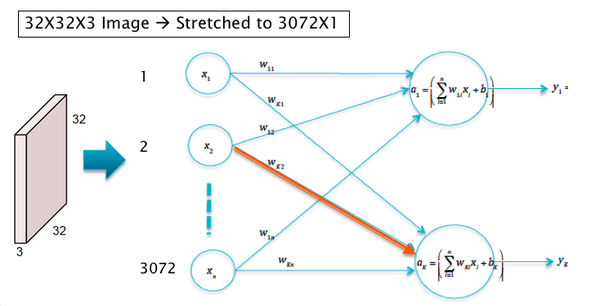In order to motivate the design of ConvNets, consider the case when the input consists of $32\times 32\times 3$ CIFAR-10 images, which have to be classified into one of ten categories. Also assume that these images are processed by a Linear Model of the type discussed in Chapter LinearLearningModels (see Figure cnn3). The logits $a_k, i\le k\le 10$ in this model are given by $$a_k = \sum_{i=1}^{3072} w_{ki} x_i + b_k,\ \ 1\le k\le 10$$ Note that the sum $\sum_{i=1}^{3072} w_{ki} x_i$ is maximized if $w_{ki} = x_i,\ 1\le i\le 3072$ under the contraint that both of these are unit vectors. Hence this lends itself to the interpretation that for each category $k$, the weights $w_{ki}, 1\le i\le 3072$ are a template or filter for the category $k$ object, which looks for images that resemble the filter itself. Hence the classification operation can be interpreted as template matching with the input image, as shown in Figure cnn4.

In :
#cnn4
nb_setup.images_hconcat(["DL_images/cnn4.png"], width=600)

Out: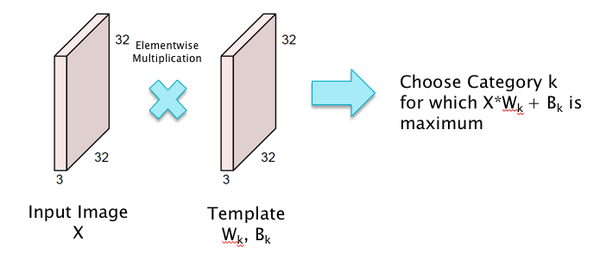This template matching interpretation of Linear Filtering naturally leads to the following idea for improving the system: Instead of using a template that tries to match the entire image, why not use smaller templates that try to match objects in smaller local portions of the image. This has the following benefits: (a) Smaller templates need a smaller filter size and thus fewer parameters, and (b) Even if the object being detected moves around the image, the same template or filter can still be used, thus leading to translational invariance. This is the main idea behind ConvNets as illustrated in Figure cnn1.

## Architecture of ConvNets¶

In :
#cnn1
nb_setup.images_hconcat(["DL_images/cnn1.png"], width=600)

Out: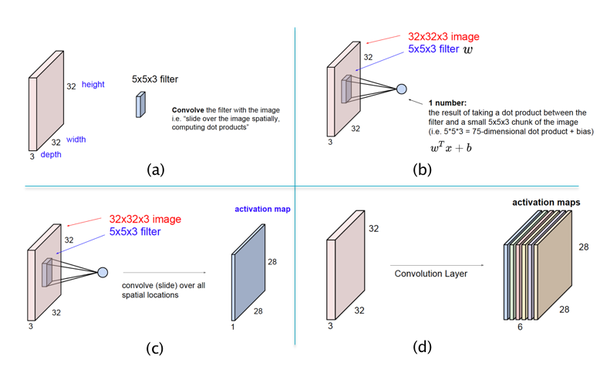The main architectural aspects of ConvNets are illustrated in parts (a) - (d) of Figure cnn1:

• Part (a) of Figure cnn1 illustrates the difference between template matching in ConvNets vs Dense Feed Forward Networks as shown in Figure cnn4: ConvNets use a template (or filter) that is smaller than the size of the image in height and width, while the depths match. In the example shown in (a), a filter of size $5\times 5\times 3$ is used for an image of size $32\times 32\times 3$.

• Part (b) shows the template matching operation in ConvNets. As shown here, the matching is done locally, for possibly overlapping patches of the image. At each position of the filter, the template matching is done using the following equation to compute the pre-activation $a$ and activation $z$:

\begin{equation} a = \sum_{i=1}^{75} w_{i} x_i + b, \end{equation}\begin{equation} z = f(a) \quad \quad (**tf**) \end{equation}
• In this equation the pixel values $(x_1,...,x_{75})$, known as the Local Receptive Field, correspond to the local image patch of size $5\times 5\times 3$ and changes as the filter is moved around (while the filter values $w_i$ and $b$ remain unchanged). Note that the filter now only has $5\times 5\times 3 + 1 = 76$ parameters, as opposed to the $32\times 32\times 3 + 1 = 3073$ parameters that were needed for the filter in Figure cnn4. Both the filter as well as the local image patch are 3-D tensors, though the multiplication in Equation (tf) uses a stretched out vectorized versions of the two tensors.

• Part (c) of the figure shows the filter being moved across the image, and at each position Equation (tf) is used to compute a new value of $z$, and this generates a matrix of size $28\times 28$. This matrix is known as an Activation Map (also called a Feature Map). This operation of sliding the filter across the image, while computing the dot product (tf) at each position, is called a convolution. Using the same Filter for all the nodes in the Activation Map implies that all nodes in the Map are tuned to detect the same feature in the Input Layer, only at different positions in the image. This leads to the conclusion that ConvNets possess the property of Translational Invariance, i.e., they are able to detect objects irrespective of their location in the image.

• Note that so far we have used a single filter which is only capable of detecting a single pattern in the input image. If we wish to detect multiple patterns, then we need multiple filters, each of which results in its own Activation Map, as shown in Part (d) of Figure cnn1. For example, Activation Map 1 may detect horizonal edges while Activation Map 2 detects vertical edges etc. As shown, a Hidden Layer in ConvNets consists of a stack of Activation Maps.

The filter in Dense Feed Forward Networks spans the entire input image, which means that it is looking for patterns that also span the entire image. However, real world images are built from smaller patterns that rarely span the entire image plane. Hence, by reducing the size of the filter, Convnets are better positioned to detect smaller shapes, which are then composed hierarchically into larger shapes and objects as we go deeper into the network. Also the Translational Invariance property ensures that the shape is detected irrespective of its location in the image plane.

## Fully Connected DLNs vs ConvNets¶

In :
#cnn2
nb_setup.images_hconcat(["DL_images/cnn2.png"], width=600)

Out: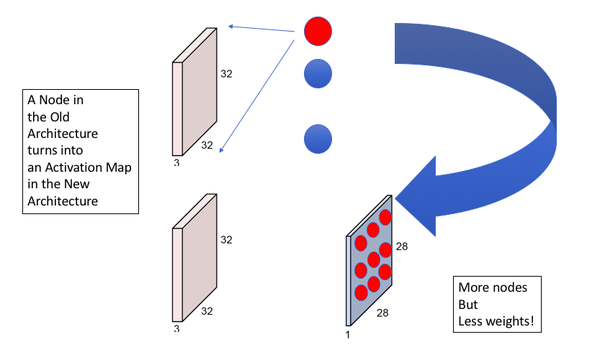Figure (cnn2) illustrates another way to contrast ConvNets with Dense Feed Forward networks. The top part of the figure shows a Dense Feed Forward Network with the input image and the nodes in the first Hidden Layer. A node in this Hidden Layer is activated if it detects a particular pattern in the image, with each node looking for a different pattern. As shown in the bottom half of the figure, with ConvNets, each of the Hidden Layer nodes is replaced by an Activation Map with multiple "sub-nodes". The activation value at each sub-node in an Activation Map is computed using a local filter which looks for the same pattern in different parts of the image. Hence in general we need as many Activation Maps in a ConvNet as there are nodes in a Hidden Layer of a Dense Feed Forward Network. This figure also illustrates that ConvNets reduce the number of weights in the model at the expense of increasing the number of nodes. The increase in nodes causes the cost of computation to go up, but this is a worthwhile tradeoff to make since the reduction in the parameters makes the model easier to train with a smaller number of training examples.

In :
#cnn5
nb_setup.images_hconcat(["DL_images/cnn5.png"], width=600)

Out: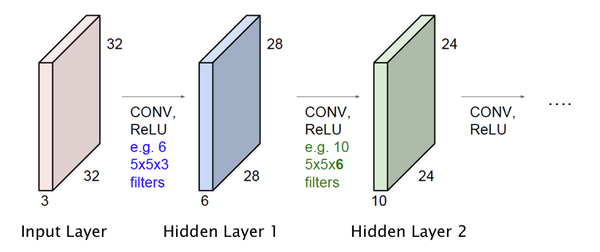So far we have only described the operation of the Input Layer and the first Hidden Layer of the ConvNet. However it is straightforward to extend this design to multiple Hidden Layers, as shown in Figure cnn5. Just as in Fully Connected Deep Feed Forward Networks, Activation Maps in the initial hidden layers detect simple shapes, which are then built upon in an hierarchical way by the later layers to detect more complex shapes. The following hyper-parameters have to be chosen with each additional layer:

• The number of Activation Maps to be used in the Hidden Layer
• The size of the Filter and the Stride size (defined below), to be used to compute the Activations.

In the example in Figure cnn5, we added a second Hidden Layer with 10 Activation Maps, that are generated using a filter of size $5\times 5\times 6$. Note that the depth of this filter is not a free variable since it has to equal to the number of Activation Maps in the previous layer.

In :
#cnn22
nb_setup.images_hconcat(["DL_images/cnn22.png"], width=900)

Out: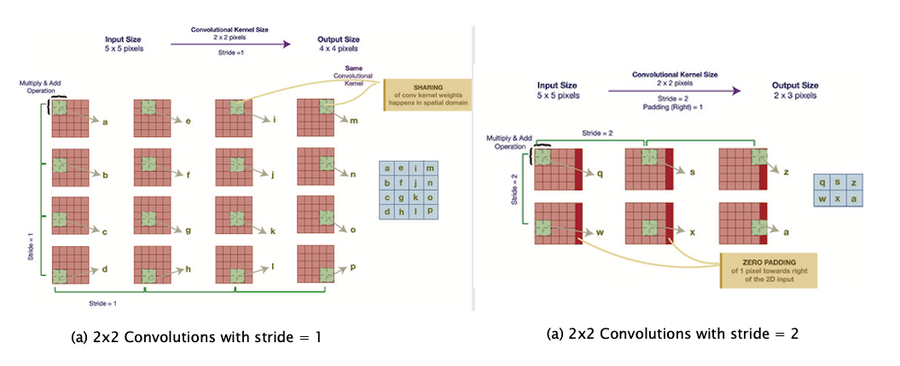The computations carried out to generate an Activation Map are shown in greater detail in Parts (a) and (b) of Figure cnn22. Part (a) shows a Filter of size $2\times 2$, operating on an input of size $5\times 5$ (without the depth dimension). In order to generate the activations, the filter is moved by one pixel at a time, horizontally and vertically, which results in an Activation Map of size $4\times 4$. As we slide the $2\times 2$ patch across the Input Layer, we compute a dot product of the activations in the Local Receptive Field with the filter weights. An important parameter used in this process is called the Stride, denoted by $S$, and is defined as the number of pixels by which the filter is moved after each computation, either horizontally or vertically. Note that the example in Part (a) corresponds to $S = 1$ while Part(b) shows the case $S = 2$. Note that for the case $S=2$, the last column in the input feature map is not accessed (shown in red). In order to remedy this, we add a an extra column of zeroes to the right, which results in an output of size $2\times 3$. This is known as Zero Padding and is discussed further later in this chapter.

Assuming that the $r^{th}$ Hidden Layer is processed using a filter of size $L\times W\times D$, note that this filter requires $L \times W \times D + 1$ parameters. However, since the same filter is used for all the nodes in the Layer $r+1$ Activation Map, it results in a huge reduction in the number of parameters needed to describe ConvNets. Since each Activation Map in Layer $r+1$ requires its own filter, so that if there are $C$ such Maps (i.e., Layer $r+1$ is of depth $C$), then the total number of parameters needed is given by $C \times (L \times W \times D + 1)$.

In order to appreciate the scale of the reduction in number of parameters due to shared filters, consider a ConvNet with Input Layer consisting of $32\times 32\times 3$ images and containing a Hidden Layer with 20 Activation Maps generated using $5 \times 5$ filters. Since each Activation Map requires $5 \times 5\times 3+1=76$ filter parameters, $76 \times 20 = 1520$ parameters are sufficient to generate the Hidden Layer. On the other hand, in a Fully Connected Deep Feed Forward architecture with $32\times 32\times 3 = 3072$ nodes in the Input Layer and 20 nodes in the Hidden Layer, a total of $3072\times 20+20 = 61,460$ parameters are needed.

## Pooling¶

In :
#convnet6
nb_setup.images_hconcat(["DL_images/convnet6.jpeg"], width=600)

Out: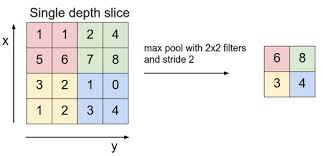In addition to the Convolution operation described above, ConvNets also feature an operation called Pooling (see Figure convnet6). Pooling usually occurs after a Convolutional Layer, and can be described as condensing the information from that layer into a smaller number of activations. As shown in the figure, Pooling involves replacing a set of activations within a region of the Activation Map (which is just like a Local Receptive Field), by a single number. Usually the maximum of the activation values in the Local Receptive Field is used for pooling (called max-pooling), but other functions can also be used, such as the $L_1$ or $L_2$ norm. Unlike in Local Receptive Fields used in Convolutions, the corresponding fields used for the Pooling operation do not overlap. Note that the addition of Pooling does not introduce any new parameters to the ConvNet and the total number of parameters in the model are reduced considerably due to this operation.

In order to understand the Pooling operation, note that the numbers in an Activation Map that results from the Convolution operation, correspond to the likelihood of whether a particular shape or pattern is present in various locations in the previous layer. By doing Pooling right after Convolution, we throw away some of that information, which is the same as saying that the network does not care about the exact location of a pattern, it only needs to know whether the pattern is present or not. It should also be mentioned that as more processing power becomes available, some modern ConvNets, such as ResNet and the Google Inception Network, no longer incorporate Pooling in their design.

In :
## Global Max Pooling

In :
#convnet29
nb_setup.images_hconcat(["DL_images/convnet29.png"], width=600)

Out: